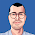## Sunday, November 1, 2015

### Vacancies in Europe

I like playing around with data from Eurostat. At this time the tools to do so are just so easy. There are tools to pull the data directly from the data base in R (eurostat package). Process it a bit using dplyr and before you know it, ggplot makes a plot.

### Data

My starting point to examine data is the database page. From there I can browse for the correct table and view its contents. Having done that, I can take the name of the table and pull that in R. The name of the vacancy database I chose (Job vacancy statistics - quarterly data (from 2001 onwards), NACE Rev. 2) is jvs_q_nace2, hence with
library(eurostat)
library(dplyr)
library(ggplot2)
library(scales)
r1 <- get_eurostat('jvs_q_nace2')
I have all packages needed and the data in R. One of the properties of the data is that everything is coded. Hence the next step is to merge the codes. The following code pulls the country codes and does a bit of post processing on the names to get them a bit nicer. Subsequently, the variously combinations of countries determined by expanding of the EU and Euro area at various time points are removed. These data have the property that they are too abundant, some data removal is needed. Finally, seasonably adjusted data is selected and all company sizes are used.
# add country names
r2 <- get_eurostat_dic('geo') %>%
mutate(.,
geo=V1,
country=V2,
country=gsub('\\(.*\$','',country),
country=gsub(' \$','',country)) %>%
select(.,geo,country) %>%
merge(.,r1) %>%
# filter countries
filter(.,
!grepl('EA.*',geo),
!grepl('EU.*',geo),
sizeclas!='Total') %>% # all company sizes
mutate(.,country=factor(country)) %>%
For other variables, it is more or less the same. get_eurostat_dic() pulls the coding and they can be merged. The text in nace is a bit long, so I shortened it.
r3 <- get_eurostat_dic('nace_r2') %>%
rename(.,
nace_r2=V1, # add NACE
nace=V2) %>%
mutate(.,
nace=substr(nace,1,110)) %>%
merge(.,r2) %>%
mutate(nace=factor(nace))

r4 <- get_eurostat_dic('indic_em') %>%
rename(.,
indic_em=V1) %>%
merge(.,r3) %>%
mutate(.,
property=factor(V2)) %>%
select(.,-V2)

### Plots

Since the data is now prepared, the next step is to plot. There are actually far too many categories in nace and a selection to be displayed is needed. If you want know what different categories are, use
nace <- select(r4,nace_r2,nace) %>% unique()
to display what each category represents. I chose to select a number of industry related categories. In addition some countries have very limited data, they are eliminated.
filter(r4,
property=='Job vacancy rate',
nace_r2 %in% c('A-S','B-E','B-S','B-F'),
!(country %in%
c('Croatia', 'Greece','Portugal',# limited years
'Switzerland')),             # limited classes
time>as.Date('01-01-2006',format='%d-%m-%Y'),
!is.na(values)) %>%
mutate(.,country=factor(country)
,nace_r2=factor(nace_r2)) %>%

ggplot(.,aes(x=time,y=values,color=nace)) +
geom_line() +
facet_wrap(  ~ country )+
ylab('Job vacancy rate')+
guides(color=guide_legend(ncol=1))+
scale_x_date(labels=date_format("%y"))+
xlab('Year')+
theme(legend.position="bottom", legend.title=element_blank())
In the plot the enormous drops for Cyprus, Czech Republic and Estonia are clearly visible. The Czech Republic is also rebounding quite steeply. UK had a smaller drop in 2008, but is now at pre-crisis job vacancy rates. In fact many countries show increases in job vacancy rate.

Getting a different display is just very easy. Below the call to get number of vacancies in education, information and communication and research. Since the number of vacancies is really dependent on country size, a logarithmic scale is chosen. The countries displayed are slightly different, it appears not all countries have all data. But the trends are similar as the previous plot.

filter(r4,
property=='Number of job vacancies',
nace_r2 %in% c('J','M','M_N','P'),
!(country %in% # limited years
c('Croatia', 'Greece','Portugal','Sweden')),
!is.na(values)) %>%
mutate(.,country=factor(country)
,nace_r2=factor(nace_r2)) %>%

ggplot(.,aes(x=time,y=values,color=nace )) +
geom_line() +
facet_wrap(  ~ country )+
ylab('Number of job vacancies')+
guides(color=guide_legend(ncol=1))+
scale_x_date(labels=date_format("%y"))+
xlab('Year')+
scale_y_log10()+
theme(legend.position="bottom", legend.title=element_blank())

#### 1 comment:

1.Thanks for your article, I didn't know about the eurostat package before, it's great.

I found that get_eurostat_dic() is not needed often. Function label_eurostat(r1) transforms all codes in data frame to long names easily and all at once.